BMW，这个来自巴伐利亚的汽车品牌到底对技术与质量有多执着？从一辆全新BMW 5系Li上可见一斑，它是工业4.0时代下诞生的汽车作品，饱含了宝马的前沿科技，给了我们耳目一新的享受和面向未来的自信。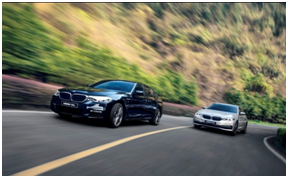然而，在我们眼睛看不到的更多地方，宝马对于细节更是执着到近乎执拗，甚至连一颗螺丝的拧紧程度都有数据可供追溯，为的就是尽可能让用户拥有更好的体验。即使这些优势并不能触目所及，宝马也会不惜投入大量精力和成本。这是宝马的经典所在，是宝马卓越驾驶乐趣的殷实内涵。

今天，就让我们来透视一辆全新BMW 5系Li，看看那些不为注意的硬实力。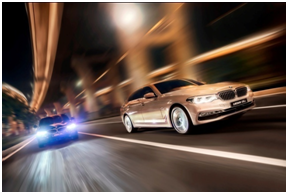智能轻量化的车身，既轻，又强

在同级细分市场中，全新5系有着更胜一筹的3108毫米超长轴距、超过5米的车身，这样一个庞然大物却会让拥有者为它的矫健身手而自豪，运动员的身体素质，车身既轻盈又结实。这一优越感的第一步基础，就来自于它的智能轻量化设计。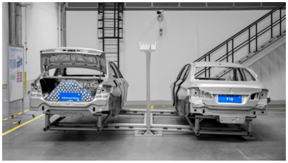在智能轻量化的设计之下，全新BMW 5系Li的整车重量比上一代减轻了最多达130公斤，相当于少了整整两位成年人的重量，自然轻快。贡献出来的好处就太多了，包括操控更敏捷、乘坐更舒适、油耗更经济等等。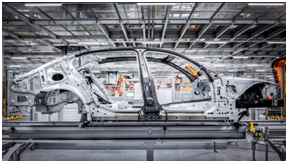单单白车身一项，也就是还没涂装、没安装底盘、动力系统、电气元件和内外饰等配置的车身状态，就比上代车型轻了50公斤（大约170瓶330毫升的矿泉水）。别看减重了，车身还比以前还更坚固、更安全了，用工程师的话来说，“那是相当的强”！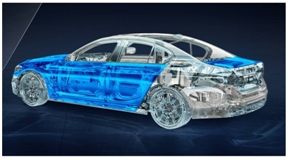最突出的就是大量的铝制部件和热成型超高强度钢的有机结合——四门两盖包括前翼子板都采用铝合金材质，在框架结构和底梁上则使用比前代更多的超高强度钢，车门上也采用了超高强度钢的加强梁，是真正的“钢筋钢骨”。在车身要求强度最高的ABC柱，采用热成型钢，它们强悍到什么地步呢？简单来说指甲盖大小的面积上可以撑起一头成年非洲象，足以给车厢内乘员强大的保护，再具象来说，承受飞机起降的航空母舰的钢板强度也远不如全新5系结实。

精益求精的底盘，或犀利，或舒适

除了大幅减重以外，宝马的工程师们同样花了非常大的力气保持车身的50:50理想配重比，在点点滴滴之处都在斤斤计较，来实现更好的动态效果。在底盘，铝制部件也得到了大幅应用，让车的重量进一步减轻。后悬则为了让运动性能所需的弹性和轻量化更均衡，特别把铝合金与特殊设计的钢制连杆相结合，让它既有铝合金的轻量化，又有钢材的韧性。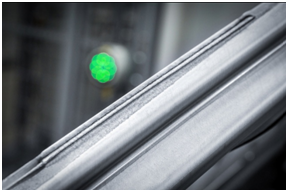全新设计的5连杆后悬结构，每一根连杆都有独立的方向，使车辆的控制更加精准。在5连杆后悬的基础上加装了空气弹簧和EDC电子减震系统。电子控制调节的VDC阻尼系统可以使全新车型的悬架软硬可调，结合可调整的弹簧系数，进一步增强了乘坐舒适性和动态驾驶体验。在操控的过程中，驾驶者能够感觉出车辆对路面反应回弹调整得极为到位，精准之余舒适感随之提升。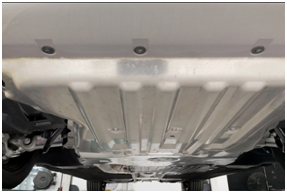全新5系的底盘响应速度极快，通过后轴上的主动稳定器，动态减震控制系统可以在行驶中不断调整悬架，让车辆平稳应对突然状况。新一代车型采用的双弹性支撑，让底盘、动力系统的连接点全部使用弹性连接，大大降低了动力输出时的振动。于是，在突然急加速、急减速时，车上乘客同样能得到非常好的乘坐舒适性和静音性。全新BMW 5系Li还在靠外的弹簧上做了减震支柱设计，降低了后桥的刚性从而提升了舒适性。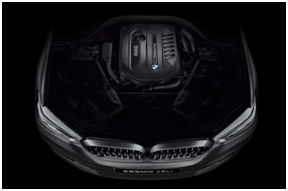澎湃的动力，要激情，也要安静

屡获国际发动机大奖的宝马B系列发动机，与改进型8速手自一体变速箱搭档，打造出黄金动力组合，既有澎湃的动力，又兼顾毫无顿挫的顺畅感，能够在行驶的各个阶段充分调动车的超强驾控能力。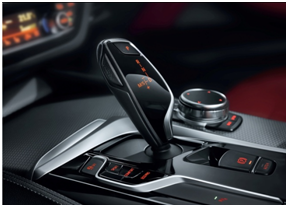在宝马B系列发动机中，我们可以列出一长串先进技术，宝马的工程师们将方方面面都琢磨得极尽细致。融合涡轮增压、第四代电子气门技术、HPI高精度缸内直喷三项发动机关键技术于一身的宝马B系列发动机，无论动力传输效率、顺畅程度、平稳性，还是油耗、发动机体积、重量、噪音水平，都达到了出色的平衡状态。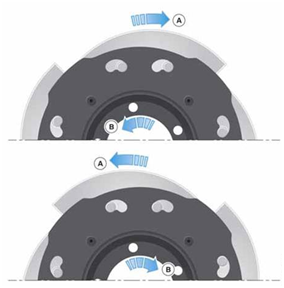改进型8速手自一体变速箱也有了很大变化。首先，在整个范围内的传动比都比原来更大，传动效率和反应速度随之提升。更重要的是，新型的带离心摆式减震器的液力变矩器的应用，抵消掉了发动机的扭转振动，使得驾驶舒适性则被大大提高。整体变速箱不但换挡更快，传动效率更胜从前，以往就广受青睐的平顺与舒适性也更出色。可以说，全新5系的静音效果是历代车型中最棒的，绝对会让用户惊喜。在全新5系上，使用了一种技术叫SYNTAK协同热声舱技术，将发动机舱、发动机与变速箱均以隔音隔热的材料来包覆，来大大减低传入座舱内的噪音和热量。如果不拆开看，很难想到隔音隔热技术已经应用到了如此地步。同时，接触式激光焊的高精密度也使得车门的密合度比以往都更好，在轮毂、车顶也集成了嵌入式的隔音板，无论开车还是坐车，你都可以安享属于自己的静谧世界。

##### 相关资讯

|分享

•已阅！握爪
•我手滑为你点赞
•128个赞！
•不明觉厉
•阅后既醉
•有钱！任性
•照片太美，我不敢看
•干货！杠杠的
•高大上
•膜拜中！
•请接受我的膝盖
•猫哥/猫妹么么哒
•神吐槽
•我只笑笑不说话
•我想静静
•窒息
##### 资讯相关车系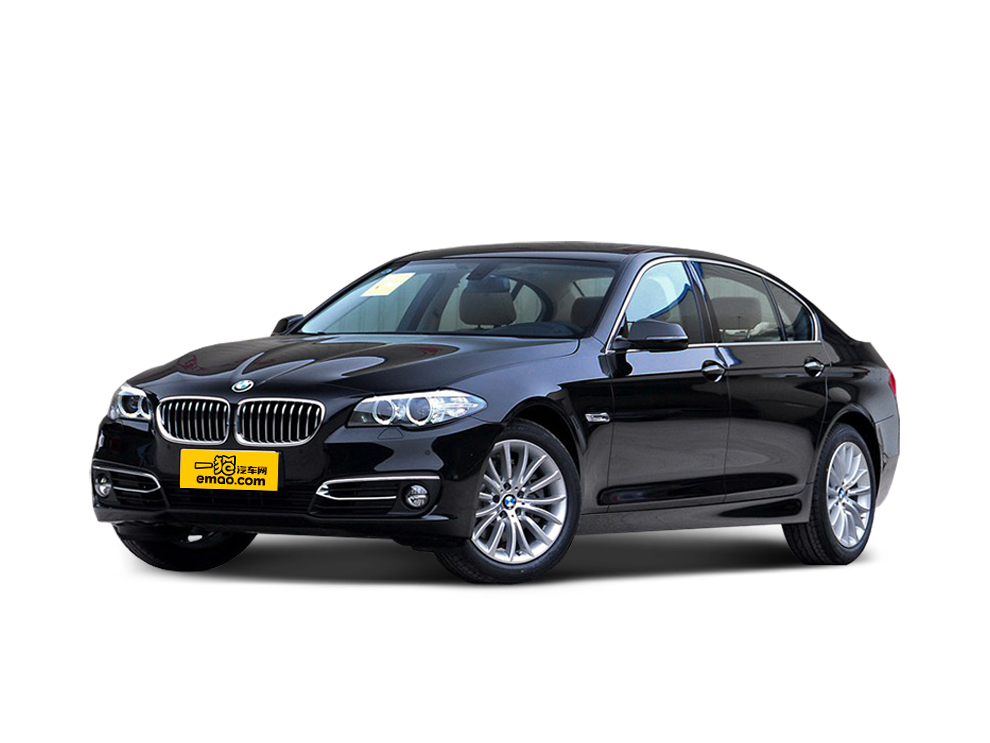##### 热度排行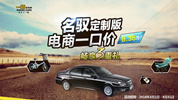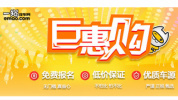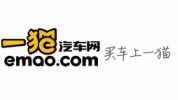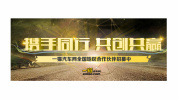• 阿斯顿·马丁
• 奥迪
• 阿尔法罗密欧
• ALPINA

• 巴博斯
• 宝骏
• 宝马
• 保时捷
• 北汽制造
• 奔驰
• 奔腾
• 本田
• 比亚迪
• 标致
• 别克
• 宾利
• 布加迪
• 北汽威旺
• 北京
• 北汽绅宝
• 北汽幻速
• 北汽新能源
• 宝沃
• 比速汽车
• 北汽道达

• 昌河
• 长安
• 长城
• 长安商用
• 成功汽车
• 长江EV
• 长安轻车型

• 大众
• 道奇
• 东风
• 东风风神
• 东风小康
• 东南
• DS
• 东风风行
• 东风风度
• 东风风光

• 法拉利
• 菲亚特
• 丰田
• 福迪
• 福特
• 福田汽车
• 福汽启腾
• 风诺

• GMC
• 广汽传祺
• 广汽吉奥
• 观致

• 哈飞
• 海格
• 海马
• 华泰
• 黄海
• 恒天
• 红旗
• 哈弗
• 华颂
• 华凯
• 华泰新能源
• 汉腾汽车

• Jeep
• 江淮
• 江铃
• 捷豹
• 金杯
• 九龙
• 吉利汽车
• 金旅
• 金龙
• 江铃集团轻汽
• 江铃集团新能源
• 君马汽车
• 捷途

• 开瑞
• 凯迪拉克
• 科尼赛克
• 克莱斯勒
• KTM
• 卡威
• 凯翼
• 康迪
• 康迪电动汽车集团

• 猎豹汽车
• 兰博基尼
• 劳斯莱斯
• 雷克萨斯
• 雷诺
• 理念
• 力帆
• 莲花汽车
• 林肯
• 铃木
• 陆风
• 路虎
• 路特斯
• 领志
• 领克
• 零跑汽车

• MG
• MINI
• 马自达
• 玛莎拉蒂
• 迈凯伦
• 摩根

• 纳智捷

• 讴歌
• 欧宝
• 欧朗
• 欧拉
• 欧尚汽车

• 帕加尼

• 奇瑞
• 启辰
• 起亚
• 前途
• 庆铃汽车

• 日产
• 荣威
• 瑞麒汽车
• 如虎
• 瑞驰

• 上汽大通
• smart
• 三菱
• 双环
• 双龙
• 斯巴鲁
• 斯柯达
• 萨博
• 思铭
• 赛麟
• SWM斯威汽车

• TESLA
• 泰卡特
• 腾势

• 威麟
• 威兹曼
• 沃尔沃
• 五菱汽车
• 五十铃
• 潍柴英致
• WEY
• 蔚来
• 威马汽车

• 现代
• 雪佛兰
• 雪铁龙
• 西雅特
• 新特汽车
• 小鹏汽车
• 新宝骏

• 野马汽车
• 一汽
• 依维柯
• 英菲尼迪
• 永源
• 驭胜

• 中华
• 中兴
• 众泰
• 知豆
• 之诺
• 正道汽车
• A
• B
• C
• D
• E
• F
• G
• H
• I
• J
• K
• L
• M
• N
• O
• P
• Q
• R
• S
• T
• U
• V
• W
• X
• Y
• Z

• 阿斯顿·马丁
• 奥迪
• 阿尔法罗密欧
• ALPINA

• 巴博斯
• 宝骏
• 宝马
• 保时捷
• 北汽制造
• 奔驰
• 奔腾
• 本田
• 比亚迪
• 标致
• 别克
• 宾利
• 布加迪
• 北汽威旺
• 北京
• 北汽绅宝
• 北汽幻速
• 北汽新能源
• 宝沃
• 比速汽车
• 北汽道达

• 昌河
• 长安
• 长城
• 长安商用
• 成功汽车
• 长江EV
• 长安轻车型

• 大众
• 道奇
• 东风
• 东风风神
• 东风小康
• 东南
• DS
• 东风风行
• 东风风度
• 东风风光

• 法拉利
• 菲亚特
• 丰田
• 福迪
• 福特
• 福田汽车
• 福汽启腾
• 风诺

• GMC
• 广汽传祺
• 广汽吉奥
• 观致

• 哈飞
• 海格
• 海马
• 华泰
• 黄海
• 恒天
• 红旗
• 哈弗
• 华颂
• 华凯
• 华泰新能源
• 汉腾汽车

• Jeep
• 江淮
• 江铃
• 捷豹
• 金杯
• 九龙
• 吉利汽车
• 金旅
• 金龙
• 江铃集团轻汽
• 江铃集团新能源
• 君马汽车
• 捷途

• 开瑞
• 凯迪拉克
• 科尼赛克
• 克莱斯勒
• KTM
• 卡威
• 凯翼
• 康迪
• 康迪电动汽车集团

• 猎豹汽车
• 兰博基尼
• 劳斯莱斯
• 雷克萨斯
• 雷诺
• 理念
• 力帆
• 莲花汽车
• 林肯
• 铃木
• 陆风
• 路虎
• 路特斯
• 领志
• 领克
• 零跑汽车

• MG
• MINI
• 马自达
• 玛莎拉蒂
• 迈凯伦
• 摩根

• 纳智捷

• 讴歌
• 欧宝
• 欧朗
• 欧拉
• 欧尚汽车

• 帕加尼

• 奇瑞
• 启辰
• 起亚
• 前途
• 庆铃汽车

• 日产
• 荣威
• 瑞麒汽车
• 如虎
• 瑞驰

• 上汽大通
• smart
• 三菱
• 双环
• 双龙
• 斯巴鲁
• 斯柯达
• 萨博
• 思铭
• 赛麟
• SWM斯威汽车

• TESLA
• 泰卡特
• 腾势

• 威麟
• 威兹曼
• 沃尔沃
• 五菱汽车
• 五十铃
• 潍柴英致
• WEY
• 蔚来
• 威马汽车

• 现代
• 雪佛兰
• 雪铁龙
• 西雅特
• 新特汽车
• 小鹏汽车
• 新宝骏

• 野马汽车
• 一汽
• 依维柯
• 英菲尼迪
• 永源
• 驭胜

• 中华
• 中兴
• 众泰
• 知豆
• 之诺
• 正道汽车
• A
• B
• C
• D
• E
• F
• G
• H
• I
• J
• K
• L
• M
• N
• O
• P
• Q
• R
• S
• T
• U
• V
• W
• X
• Y
• Z

• 阿斯顿·马丁
• 奥迪
• 阿尔法罗密欧
• ALPINA

• 巴博斯
• 宝骏
• 宝马
• 保时捷
• 北汽制造
• 奔驰
• 奔腾
• 本田
• 比亚迪
• 标致
• 别克
• 宾利
• 布加迪
• 北汽威旺
• 北京
• 北汽绅宝
• 北汽幻速
• 北汽新能源
• 宝沃
• 比速汽车
• 北汽道达

• 昌河
• 长安
• 长城
• 长安商用
• 成功汽车
• 长江EV
• 长安轻车型

• 大众
• 道奇
• 东风
• 东风风神
• 东风小康
• 东南
• DS
• 东风风行
• 东风风度
• 东风风光

• 法拉利
• 菲亚特
• 丰田
• 福迪
• 福特
• 福田汽车
• 福汽启腾
• 风诺

• GMC
• 广汽传祺
• 广汽吉奥
• 观致

• 哈飞
• 海格
• 海马
• 华泰
• 黄海
• 恒天
• 红旗
• 哈弗
• 华颂
• 华凯
• 华泰新能源
• 汉腾汽车

• Jeep
• 江淮
• 江铃
• 捷豹
• 金杯
• 九龙
• 吉利汽车
• 金旅
• 金龙
• 江铃集团轻汽
• 江铃集团新能源
• 君马汽车
• 捷途

• 开瑞
• 凯迪拉克
• 科尼赛克
• 克莱斯勒
• KTM
• 卡威
• 凯翼
• 康迪
• 康迪电动汽车集团

• 猎豹汽车
• 兰博基尼
• 劳斯莱斯
• 雷克萨斯
• 雷诺
• 理念
• 力帆
• 莲花汽车
• 林肯
• 铃木
• 陆风
• 路虎
• 路特斯
• 领志
• 领克
• 零跑汽车

• MG
• MINI
• 马自达
• 玛莎拉蒂
• 迈凯伦
• 摩根

• 纳智捷

• 讴歌
• 欧宝
• 欧朗
• 欧拉
• 欧尚汽车

• 帕加尼

• 奇瑞
• 启辰
• 起亚
• 前途
• 庆铃汽车

• 日产
• 荣威
• 瑞麒汽车
• 如虎
• 瑞驰

• 上汽大通
• smart
• 三菱
• 双环
• 双龙
• 斯巴鲁
• 斯柯达
• 萨博
• 思铭
• 赛麟
• SWM斯威汽车

• TESLA
• 泰卡特
• 腾势

• 威麟
• 威兹曼
• 沃尔沃
• 五菱汽车
• 五十铃
• 潍柴英致
• WEY
• 蔚来
• 威马汽车

• 现代
• 雪佛兰
• 雪铁龙
• 西雅特
• 新特汽车
• 小鹏汽车
• 新宝骏

• 野马汽车
• 一汽
• 依维柯
• 英菲尼迪
• 永源
• 驭胜

• 中华
• 中兴
• 众泰
• 知豆
• 之诺
• 正道汽车
• A
• B
• C
• D
• E
• F
• G
• H
• I
• J
• K
• L
• M
• N
• O
• P
• Q
• R
• S
• T
• U
• V
• W
• X
• Y
• Z